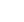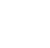# Linear Algebra IV: Orthogonality & Symmetric Matrices and the SVD

## Description

In the first part of this course you will explore methods to compute an approximate solution to an inconsistent system of equations that have no solutions. Our overall approach is to center our algorithms on the concept of distance. To this end, you will first tackle the ideas of distance and orthogonality in a vector space. You will then apply orthogonality to identify the point within a subspace that is nearest to a point outside of it. This has a central role in the understanding of solutions to inconsistent systems. By taking the subspace to be the column space of a matrix, you will develop a method for producing approximate (“least-squares”) solutions for inconsistent systems. You will then explore another application of orthogonal projections: creating a matrix factorization widely used in practical applications of linear algebra. The remaining sections examine some of the many least-squares problems that arise in applications, including the least squares procedure with more general polynomials and functions. This course then turns to symmetric matrices. arise more often in applications, in one way or another, than any other major class of matrices. You will construct the diagonalization of a symmetric matrix, which gives a basis for the remainder of the course.

## Specific details

Category of Education Physical Science

## University

Georgia Institute of Technology

There are no comments posted here yetinfo@educom.net
 About Terms and ConditionsPrivacy PolicyRankingsAccreditations